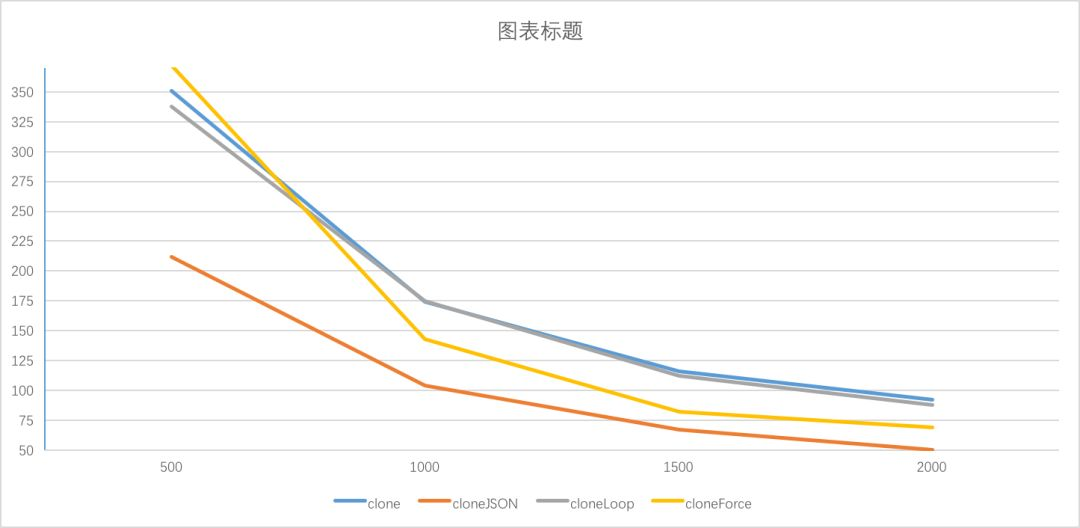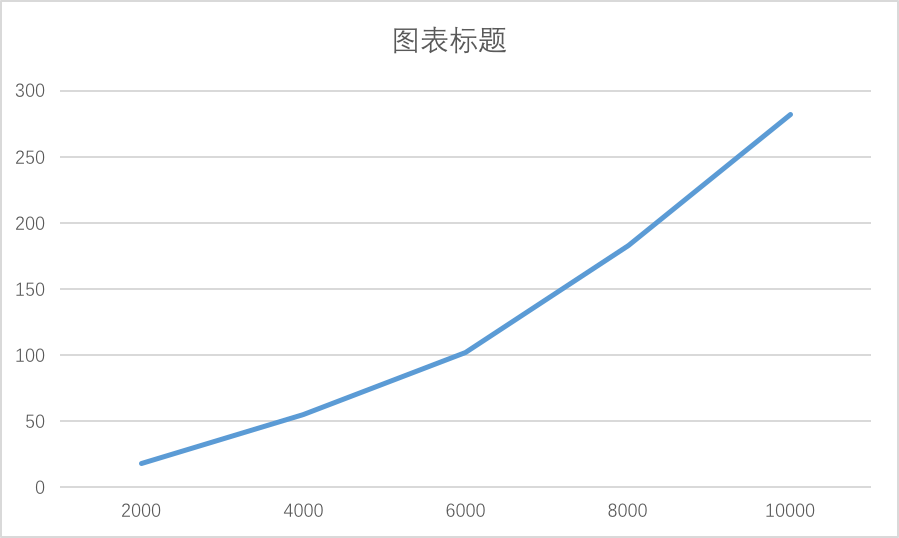# 深拷贝的终极探索

#### 深拷贝 VS 浅拷贝

``````// 基本类型
var a = 1;
var b = a;
a = 2;
console.log(a, b); // 2, 1    a b指向不同的数据
// 引用类型指向同一份数据
var a = {c: 1};
var b = a;
a.c = 2;
console.log(a.c, b.c); // 2, 2 全是2   a b指向同一份数据``````

``````function shallowClone(source) {
var target = {};
for(var i in source) {
if (source.hasOwnProperty(i)) {
target[i] = source[i];
}
}

return target;
}``````

#### 最简单的深拷贝

``````var a1 = {
b:  {
c: {d: 1}
}
};``````

``````function clone(source) {
var target = {};
for(var i in source) {
if (source.hasOwnProperty(i)) {
if (typeof source[i] === 'object') {
target[i] = clone(source[i]); // 注意这里
} else {
target[i] = source[i];
}
}
}

return target;
}``````

• 没有对参数做检验
• 判断是否对象的逻辑不够严谨
• 没有考虑数组的兼容

(⊙o⊙)，下面我们来看看各个问题的解决办法，首先我们需要抽象一个判断对象的方法，其实比较常用的判断对象的方法如下，其实下面的方法也有问题，但如果能够回答上来那就非常不错了，如果完美的解决办法感兴趣，不妨看看这里吧

``````function isObject(x) {
return Object.prototype.toString.call(x) === '[object Object]';
}``````

``````function clone(source) {
if (!isObject(source)) return source;

// xxx
}``````

``````function createData(deep, breadth) {
var data = {};
var temp = data;

for (var i = 0; i < deep; i++) {
temp = temp['data'] = {};
for (var j = 0; j < breadth; j++) {
temp[j] = j;
}
}

return data;
}
createData(1, 3); // 1层深度，每层有3个数据 {data: {0: 0, 1: 1, 2: 2}}
createData(3, 0); // 3层深度，每层有0个数据 {data: {data: {data: {}}}}``````

``````clone(createData(1000)); // ok
clone(createData(10000)); // Maximum call stack size exceeded
clone(createData(10, 100000)); // ok 广度不会溢出``````

``````var a = {};
a.a = a;
clone(a) // Maximum call stack size exceeded 直接死循环了有没有，/(ㄒoㄒ)/~~``````

#### 一行代码的深拷贝

``````function cloneJSON(source) {
return JSON.parse(JSON.stringify(source));
}``````

``cloneJSON(createData(10000)); // Maximum call stack size exceeded``

``````var a = {};
a.a = a;
cloneJSON(a) // Uncaught TypeError: Converting circular structure to JSON``````

#### 破解递归爆栈

``````var a = {
a1: 1,
a2: {
b1: 1,
b2: {
c1: 1
}
}
}``````

``````  a
/   \
a1   a2
|    / \
1   b1 b2
|   |
1  c1
|
1``````

``````function cloneLoop(x) {
const root = {};

// 栈
const loopList = [
{
parent: root,
key: undefined,
data: x,
}
];

while(loopList.length) {
// 深度优先
const node = loopList.pop();
const parent = node.parent;
const key = node.key;
const data = node.data;

// 初始化赋值目标，key为undefined则拷贝到父元素，否则拷贝到子元素
let res = parent;
if (typeof key !== 'undefined') {
res = parent[key] = {};
}

for(let k in data) {
if (data.hasOwnProperty(k)) {
if (typeof data[k] === 'object') {
// 下一次循环
loopList.push({
parent: res,
key: k,
data: data[k],
});
} else {
res[k] = data[k];
}
}
}
}

return root;
}``````

#### 破解循环引用

``````var b = {};
var a = {a1: b, a2: b};
a.a1 === a.a2 // true
var c = clone(a);
c.a1 === c.a2 // false``````

find 是抽象的一个函数，其实就是遍历 uniqueList

``````// 保持引用关系
function cloneForce(x) {
// =============
const uniqueList = []; // 用来去重
// =============

let root = {};

// 循环数组
const loopList = [
{
parent: root,
key: undefined,
data: x,
}
];

while(loopList.length) {
// 深度优先
const node = loopList.pop();
const parent = node.parent;
const key = node.key;
const data = node.data;

// 初始化赋值目标，key为undefined则拷贝到父元素，否则拷贝到子元素
let res = parent;
if (typeof key !== 'undefined') {
res = parent[key] = {};
}

// =============
// 数据已经存在
let uniqueData = find(uniqueList, data);
if (uniqueData) {
parent[key] = uniqueData.target;
continue; // 中断本次循环
}

// 数据不存在
// 保存源数据，在拷贝数据中对应的引用
uniqueList.push({
source: data,
target: res,
});
// =============

for(let k in data) {
if (data.hasOwnProperty(k)) {
if (typeof data[k] === 'object') {
// 下一次循环
loopList.push({
parent: res,
key: k,
data: data[k],
});
} else {
res[k] = data[k];
}
}
}
}

return root;
}
function find(arr, item) {
for(let i = 0; i < arr.length; i++) {
if (arr[i].source === item) {
return arr[i];
}
}

return null;
}``````

``````var b = {};
var a = {a1: b, a2: b};
a.a1 === a.a2 // truevar c = cloneForce(a);
c.a1 === c.a2 // true``````

``````var a = {};
a.a = a;
cloneForce(a)``````

#### 性能对比

``````function runTime(fn, time) {
var stime = Date.now();
var count = 0;
while(Date.now() - stime < time) {
fn();
count++;
}

return count;
}
runTime(function () { clone(createData(500, 1)) }, 2000);``````

500351212338372
1000174104175143
15001166711282
200092508869

• 随着深度变小，相互之间的差异在变小
• clone 和 cloneLoop 的差别并不大
• cloneLoop > cloneForce > cloneJSON• clone 时间 = 创建递归函数 + 每个对象处理时间
• cloneJSON 时间 = 循环检测 + 每个对象处理时间 * 2 （递归转字符串 + 递归解析）
• cloneLoop 时间 = 每个对象处理时间
• cloneForce 时间 = 判断对象是否缓存中 + 每个对象处理时间

cloneJSON 的速度只有 clone 的 50%，很容易理解，因为其会多进行一次递归时间

cloneForce 由于要判断对象是否在缓存中，而导致速度变慢，我们来计算下判断逻辑的时间复杂度，假设对象的个数是 n，则其时间复杂度为 O(n2)，对象的个数越多，cloneForce 的速度会越慢

``1 + 2 + 3 ... + n = n^2/2 - 1``

013400327214292989

• 随着对象的增多，cloneForce 的性能低下凸显
• cloneJSON 的性能也大打折扣，这是因为循环检测占用了很多时间
• cloneLoop 的性能高于 clone，可以看出递归新建函数的时间和循环对象比起来可以忽略不计

``````var data1 = createData(2000, 0);
var data2 = createData(4000, 0);
var data3 = createData(6000, 0);
var data4 = createData(8000, 0);
var data5 = createData(10000, 0);
cloneForce(data1)
cloneForce(data2)
cloneForce(data3)
cloneForce(data4)
cloneForce(data5)``````#### 总结

clonecloneJSONcloneLoopcloneForce

@颜海镜，知名技术博主，开源达人，《React 状态管理与同构实战》作者

## 本文链接：

http://godrry.com/archives/the-ultimate-exploration-of-deep-copy-1.html
1 + 5 =